Download Presentation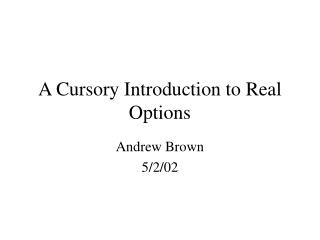A Cursory Introduction to Real Options

# A Cursory Introduction to Real Options - PowerPoint PPT Presentation

A Cursory Introduction to Real Options. Andrew Brown 5/2/02. Background. Real Options Analysis (ROA) was developed as a method to find the value of projects and assets more accurately then earlier methods such as Discounted Cash Flows (DCF) and Decision Tree Analysis (DTA).I am the owner, or an agent authorized to act on behalf of the owner, of the copyrighted work described.
Download Presentation## A Cursory Introduction to Real Options

An Image/Link below is provided (as is) to download presentation

Download Policy: Content on the Website is provided to you AS IS for your information and personal use and may not be sold / licensed / shared on other websites without getting consent from its author.While downloading, if for some reason you are not able to download a presentation, the publisher may have deleted the file from their server.

- - - - - - - - - - - - - - - - - - - - - - - - - - E N D - - - - - - - - - - - - - - - - - - - - - - - - - -
Presentation Transcript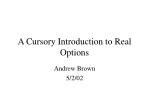### A Cursory Introduction to Real Options

Andrew Brown

5/2/02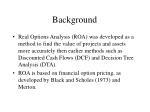Background
• Real Options Analysis (ROA) was developed as a method to find the value of projects and assets more accurately then earlier methods such as Discounted Cash Flows (DCF) and Decision Tree Analysis (DTA).
• ROA is based on financial option pricing, as developed by Black and Scholes (1973) and Merton.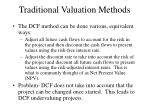• The DCF method can be done various, equivalent ways:
• Adjust all future cash flows to account for the risk in the project and then discount the cash flows to present values using the risk-free interest rate.
• Adjust the discount rate to take into account the risk of the project and discount all future cash flows to present values using the risk-adjusted interest rates. This is what is commonly thought of as Net Present Value (NPV).
• Problem- DCF does not take into account that the project can be changed once started. This leads to DCF undervaluing projects.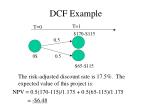T=1

T=0

\$170-\$115

0.5

0\$

0.5

\$65-\$115

DCF Example

The risk-adjusted discount rate is 17.5%. The expected value of this project is:

NPV = 0.5(170-115)/1.175 + 0.5(65-115)/1.175

= -\$6.48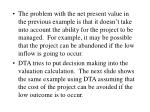The problem with the net present value in the previous example is that it doesn’t take into account the ability for the project to be managed. For example, it may be possible that the project can be abandoned if the low inflow is going to occur.
• DTA tries to put decision making into the valuation calculation. The next slide shows the same example using DTA assuming that the cost of the project can be avoided if the low outcome is to occur.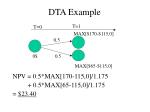T=1

T=0

MAX[\$170-\$115,0]

0.5

0\$

0.5

MAX[\$65-\$115,0]

DTA Example

NPV = 0.5*MAX[170-115,0]/1.175

+ 0.5*MAX[65-115,0]/1.175

= \$23.40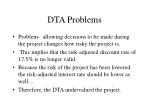DTA Problems
• Problem- allowing decisions to be made during the project changes how risky the project is.
• This implies that the risk-adjusted discount rate of 17.5% is no longer valid.
• Because the risk of the project has been lowered the risk-adjusted interest rate should be lower as well…
• Therefore, the DTA undervalued the project.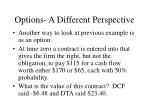Options- A Different Perspective
• Another way to look at previous example is as an option.
• At time zero a contract is entered into that gives the firm the right, but not the obligation, to pay \$115 for a cash flow worth either \$170 or \$65, each with 50% probability.
• What is the value of this contract? DCF said -\$6.48 and DTA said \$23.40.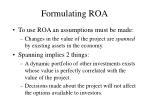Formulating ROA
• To use ROA an assumptions must be made:
• Changes in the value of the project are spanned by existing assets in the economy.
• Spanning implies 2 things:
• A dynamic portfolio of other investments exists whose value is perfectly correlated with the value of the project.
• Decisions made about the project will not affect the options available to investors.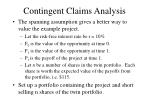Contingent Claims Analysis
• The spanning assumption gives a better way to value the example project.
• Let the risk-free interest rate be r = 10%
• F0 is the value of the opportunity at time 0.
• F1 is the value of the opportunity at time 1.
• P1 is the payoff of the project at time 1.
• Let n be a number of shares in the twin portfolio. Each share is worth the expected value of the payoffs from the portfolio, i.e. \$115.
• Set up a portfolio containing the project and short selling n shares of the twin portfolio.The value of this portfolio at time zero is:

V0 = F0 - 115*n

At time one the value is:

V1 = F1 – n* P1

= (170-65) – 170n OR

= (0) – 65n

The return will be risk free if V1 is always the same… (170-65) – 170n = 0 - 65n

Solving for n gives: n = 0.524

This gives the value of V1 to be: -\$34.08The portfolio is risk free and should return the risk free interest rate, minus the cost of the short position:

Return = V1 - V0 - .10*115*n

= (-34.08) – (F0 - 115*0.524) – 1.15*0.524

= 25.58 - F0

The return must be equal to 10% of the initial cost, i.e.,

25.58 - F0 = 0.1(F0 - 115*0.524)

F0 = \$28.73

So, ROA gives an even greater value to the project then DCF (-\$6.48) and DTA (\$23.40)Real Options Analysis
• There are three ways to evaluate real options:
• Black Scholes
• Binomial Trees
• Monte Carlo AnalysisBlack-Scholes
• To use the Black-Scholes equation to price a financial option, 5 values are needed. Listed below are the 5 financial values needed and their ROA equivalents:When Black Scholes Works
• Good for “one shot” projects.
• Lognormal distribution assumed
• No interaction between various options
• Only one source of uncertainty
• Only one maturity date

Some of these limitations can be avoided by re-deriving or modifying the Black Scholes equation.Monte Carlo Simulation
• Repeat the following a bunch of times:
• Draw random numbers for each random variable.
• Decide what choice would be made based on those number.
• Calculate the NPV
• Average the NPV over all of the runs to get an approximation of the value of the project.
• DCF would be the same as always making the same choice above, regardless of the drawn random numbers.
• The value of the real option is the difference between the DCF and the simulation as the number of runs goes to infinity, i.e., Average NPV = DCF + RealOptionWhen to use Monte Carlo
• There are multiple sources of uncertainty.
• Can use distributions besides lognormal.
• Monte Carlo simulation is slow and computationally expensive.Binomial Trees

p is the risk neutral probability that the random value goes up in a period

u is the multiplier the value goes up by.

d is the multiplied the value goes down by

Rf- per period risk free rate

T/N – number of periods

p

V*u*u

V*u

p

1-p

V*u*d=V*d*u=V

V

p

1-p

V*d*d

V*d

1-pBinomial Trees
• Can handle multiple options
• Assumes lognormal distribution
• Multiple dates for the different options to mature.
• Handles only one source of uncertainty.
• Is the same as Black Scholes as the number of intervals, N, goes to infinity.